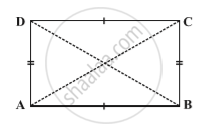Share

# Some Special Parallelograms - Concept of a Rectangle

#### description

• Property: The diagonals of a rectangle are of equal length

#### notes1) The opposite angles of a rectangle are equal.
2) The opposite sides of a rectangle are parallel to each other.
3) The adjacent angles of a rectangle ae supplementary , and
4) The diagonals of a rectangle bisect each other.

Property: The diagonals of a rectangle are of equal length.Given : ABCD is a rectangle. AC and BD are diagonals.
To prove : AC = BD
ABCD is a rectangle. Therefore it is a parallelogram.
∠A = 90°
AD = BC ,    ∠A = 90°
AD || BC & AB is transversal.
∠A + ∠B = 180°    ..(interior angles)
90° + ∠B = 180°
∠B = 90°
In ∆ ABC & ∆ ABD,
AB = AB  ..(common)
∆ ABC  ≅  ∆ ABD ,    ...(SAS rule )

### Shaalaa.com

Property: The diagonals of a rectangle are of equal length. [00:03:23]
S
0%

S